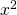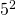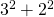Private GMAT Tutoring in NYC & Online

For which of the following functions is f(a+b) = f(b) + f(a) for all positive numbers a and b?

A. f(x) =B. f(x) = x+1
C. f(x) = √x
D. f(x) = 2/x
E. f(x) = -3x

Deja vu! Here's another function question from the GMAT prep. If you want to check out another solution for a functions question before tackling this one go here: For which of the following functions f(x)=f(1-x) and here's another extremely challenging GMAT function question that's a bit different and more on the puzzle side of things: For every positive even integer n the function h(n) is defined to be the product

Again, function questions generally look harder than they are. The first read is usually confusing. Just keep in mind that the premise is simple. For this one: find the answer choice for which plugging in a + b into the function is the same as plugging in a then plugging in b and summing the outcome. Let's just go through the answer choices. I would pick values for a and b. Let's go for a = 2, b = 3. Just a recap we want f(2 + 3) = f(2) + f(3). You can find a video explanation here: For which of the following functions is f(a+b) = f(b) + f(a) for all positive numbers a and b?

Also, for a more in depth discussion of the topic here's our main page for GMAT Functions.

A. f(x) =vs25 vs 13

B. f(x) = x+1

5 + 1 vs (2+1) + (3+1)

6 vs 7

C. f(x) = √x

√5 vs. √2 + √3

√5 is less than 3. √2 is about 1.4, √4 is about 1.7 so their sum is more than 3.

D. f(x) = 2/x

2/5 vs 2/2 + 2/3

The right side is clearly bigger.

E. f(x) = -3x

-3(5) vs -3(2) + -3(3)

-15 = -15# CONTACT

Atlantic GMAT

405 East 51st St.

NY, NY 10022

(347) 669-3545

info@AtlanticGMAT.com